In circuit shown below, the resistances are given in ohms and the battery is assumed ideal with emf equal to 3 volt. The voltage across the resistance R4 is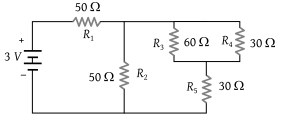(1) 0.4 V

(2) 0.6 V

(3) 1.2 V

(4) 1.5 V

Concept Questions :-

Combination of resistors
High Yielding Test Series + Question Bank - NEET 2020

Difficulty Level:

If you are provided three resistances 2 Ω, 3 Ω and 6 Ω. How will you connect them so as to obtain the equivalent resistance of 4 Ω

(1)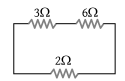(2)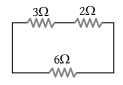(3)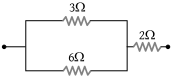(4) None of these

Concept Questions :-

Combination of resistors
High Yielding Test Series + Question Bank - NEET 2020

Difficulty Level:

The equivalent resistance and potential difference between A and B for the circuit is respectively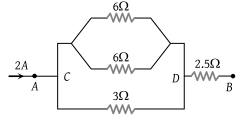(1) 4 Ω, 8 V

(2) 8 Ω, 4 V

(3) 2 Ω, 2 V

(4) 16 Ω, 8 V

Concept Questions :-

Combination of resistors
High Yielding Test Series + Question Bank - NEET 2020

Difficulty Level:

Five equal resistances each of resistance R are connected as shown in the figure. A battery of V volts is connected between A and B. The current flowing in AFCEB will be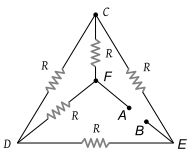(1) $\frac{3V}{R}$

(2) $\frac{V}{R}$

(3) $\frac{V}{2R}$

(4) $\frac{2V}{R}$

Concept Questions :-

Combination of resistors
High Yielding Test Series + Question Bank - NEET 2020

Difficulty Level:

For the network shown in the figure the value of the current i is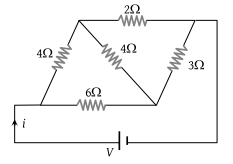(1) $\frac{9V}{35}$

(2) $\frac{5V}{18}$

(3) $\frac{5V}{9}$

(4) $\frac{18V}{5}$

Concept Questions :-

Combination of resistors
High Yielding Test Series + Question Bank - NEET 2020

Difficulty Level:

When a wire of uniform cross-section a, length l and resistance R is bent into a complete circle, the resistance between any two of diametrically opposite points will be :

(1) $\frac{R}{4}$

(2) $\frac{R}{8}$

(3) 4R

(4) $\frac{R}{2}$

Concept Questions :-

Combination of resistors
High Yielding Test Series + Question Bank - NEET 2020

Difficulty Level:

In the circuit given E = 6.0 V, R1 = 100 ohms, R2 = R3 = 50 ohms, R4 = 75 ohms. The equivalent resistance of the circuit, in ohms, is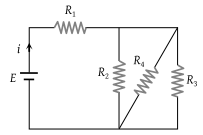(1) 11.875

(2) 26.31

(3) 118.75

(4) None of these

Concept Questions :-

Combination of resistors
High Yielding Test Series + Question Bank - NEET 2020

Difficulty Level:

By using only two resistance coils-singly, in series, or in parallel one should be able to obtain resistances of 3, 4, 12, and 16 ohms. The separate resistances of the coil are :

(1) 3 and 4

(2) 4 and 12

(3) 12 and 16

(4) 16 and 3

Concept Questions :-

Combination of resistors
High Yielding Test Series + Question Bank - NEET 2020

Difficulty Level:

In the given circuit, the voltmeter records 5 volts. The resistance of the voltmeter in ohms is :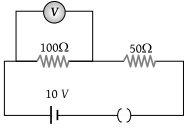(1) 200

(2) 100

(3) 10

(4) 50

Concept Questions :-

Kirchoff's voltage law
High Yielding Test Series + Question Bank - NEET 2020

Difficulty Level:

In the adjoining circuit, the battery E1 has an e.m.f. of 12 volts and zero internal resistance while the battery E has an e.m.f. of 2 volts. If the galvanometer G reads zero, then the value of the resistance X in ohm is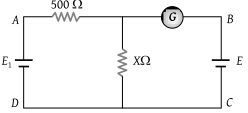(1) 10

(2) 100

(3) 500

(4) 200

Concept Questions :-

Grouping of cells